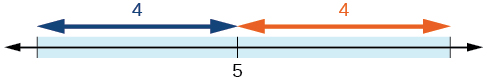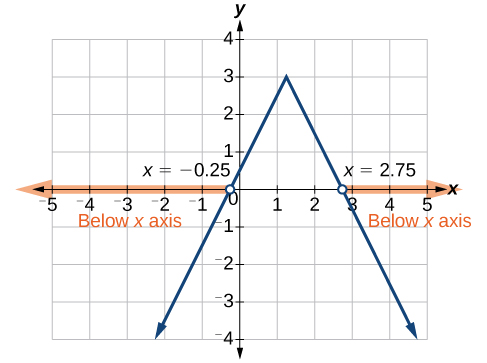## Solving Absolute Value Inequalities

As we know, the absolute value of a quantity is a positive number or zero. From the origin, a point located at $\left(-x,0\right)$ has an absolute value of $x$, as it is x units away. Consider absolute value as the distance from one point to another point. Regardless of direction, positive or negative, the distance between the two points is represented as a positive number or zero.

An absolute value inequality is an equation of the form

$|A|<B,|A|\le B,|A|>B,\text{or }|A|\ge B$,

Where A, and sometimes B, represents an algebraic expression dependent on a variable x. Solving the inequality means finding the set of all $x$ values that satisfy the problem. Usually this set will be an interval or the union of two intervals and will include a range of values.

There are two basic approaches to solving absolute value inequalities: graphical and algebraic. The advantage of the graphical approach is we can read the solution by interpreting the graphs of two equations. The advantage of the algebraic approach is that solutions are exact, as precise solutions are sometimes difficult to read from a graph.

Suppose we want to know all possible returns on an investment if we could earn some amount of money within $200 of$600. We can solve algebraically for the set of x-values such that the distance between $x$ and 600 is less than 200. We represent the distance between $x$ and 600 as $|x - 600|$, and therefore, $|x - 600|\le 200$ or

$\begin{array}{c}-200\le x - 600\le 200\\ -200+600\le x - 600+600\le 200+600\\ 400\le x\le 800\end{array}$

This means our returns would be between $400 and$800.

To solve absolute value inequalities, just as with absolute value equations, we write two inequalities and then solve them independently.

### A General Note: Absolute Value Inequalities

For an algebraic expression X, and $k>0$, an absolute value inequality is an inequality of the form

$\begin{array}{l}|X|< k\text{ is equivalent to }-k< X< k\hfill \\ |X|> k\text{ is equivalent to }X< -k\text{ or }X> k\hfill \end{array}$

These statements also apply to $|X|\le k$ and $|X|\ge k$.

### Example 9: Determining a Number within a Prescribed Distance

Describe all values $x$ within a distance of 4 from the number 5.

### Solution

We want the distance between $x$ and 5 to be less than or equal to 4. We can draw a number line, such as in Figure 4, to represent the condition to be satisfied.Figure 4

The distance from $x$ to 5 can be represented using an absolute value symbol, $|x - 5|$. Write the values of $x$ that satisfy the condition as an absolute value inequality.

$|x - 5|\le 4$

We need to write two inequalities as there are always two solutions to an absolute value equation.

$\begin{array}{lll}x - 5\le 4\hfill & \text{and}\hfill & x - 5\ge -4\hfill \\ x\le 9\hfill & \hfill & x\ge 1\hfill \end{array}$

If the solution set is $x\le 9$ and $x\ge 1$, then the solution set is an interval including all real numbers between and including 1 and 9.

So $|x - 5|\le 4$ is equivalent to $\left[1,9\right]$ in interval notation.

### Try It 9

Describe all x-values within a distance of 3 from the number 2.

Solution

### Example 10: Solving an Absolute Value Inequality

Solve $|x - 1|\le 3$.

### Solution

$\begin{array}{l}|x - 1|\le 3\hfill \\ \hfill \\ -3\le x - 1\le 3\hfill \\ \hfill \\ -2\le x\le 4\hfill \\ \hfill \\ \left[-2,4\right]\hfill \end{array}$

### Example 11: Using a Graphical Approach to Solve Absolute Value Inequalities

Given the equation $y=-\frac{1}{2}|4x - 5|+3$, determine the x-values for which the y-values are negative.

### Solution

We are trying to determine where $y<0$, which is when $-\frac{1}{2}|4x - 5|+3<0$. We begin by isolating the absolute value.

$\begin{array}{ll}-\frac{1}{2}|4x - 5|< -3\hfill & \text{Multiply both sides by -2, and reverse the inequality}.\hfill \\ |4x - 5|> 6\hfill & \hfill \end{array}$

Next, we solve for the equality $|4x - 5|=6$.

$\begin{array}{lll}4x - 5=6\hfill & \hfill & 4x - 5=-6\hfill \\ 4x=11\hfill & \text{or}\hfill & 4x=-1\hfill \\ x=\frac{11}{4}\hfill & \hfill & x=-\frac{1}{4}\hfill \end{array}$

Now, we can examine the graph to observe where the y-values are negative. We observe where the branches are below the x-axis. Notice that it is not important exactly what the graph looks like, as long as we know that it crosses the horizontal axis at $x=-\frac{1}{4}$ and $x=\frac{11}{4}$, and that the graph opens downward.Figure 5

### Try It 10

Solve $-2|k - 4|\le -6$.

Solution